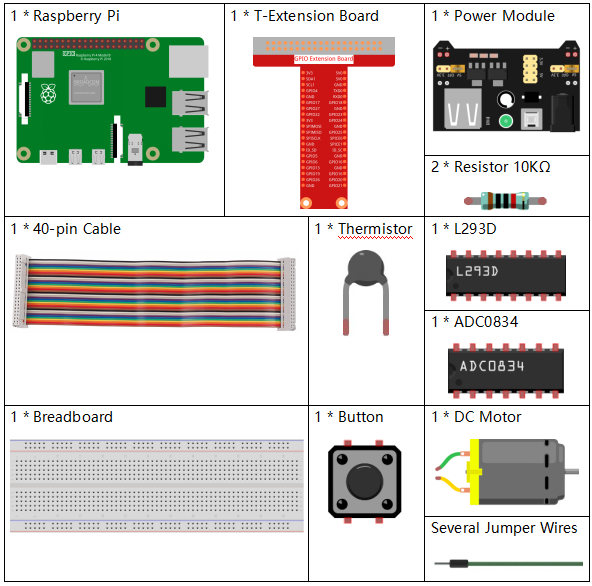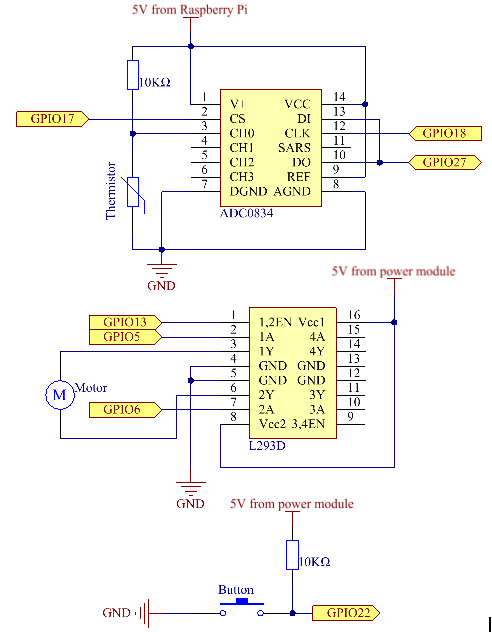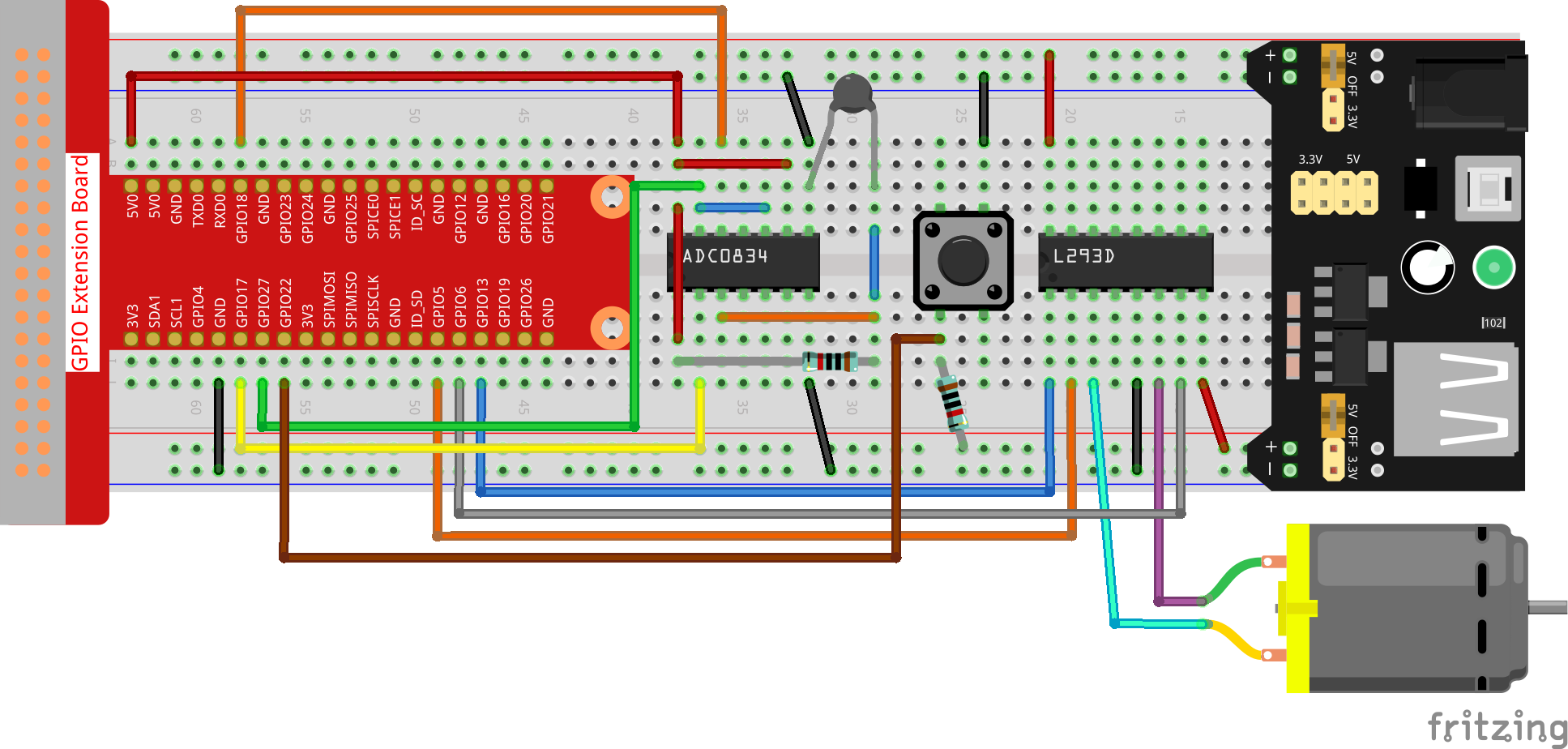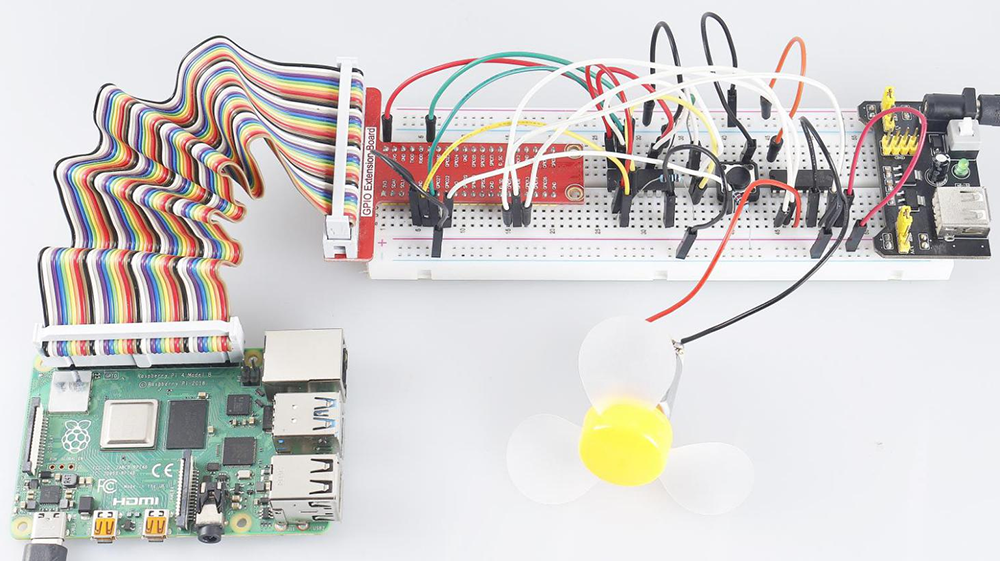# 4.1.10 Smart Fan¶

## Introduction¶

In this project, we will use motors, buttons and thermistors to make a manual + automatic smart fan whose wind speed is adjustable.

## Required Components¶

In this project, we need the following components.Name

ITEMS IN THIS KIT

Raphael Kit

337

Raphael Kit

COMPONENT INTRODUCTION

GPIO Extension Board

Jumper Wires

Resistor

Power Supply Module

-

Thermistor

L293D

-

-

Button

DC Motor

## Schematic Diagram¶

 T-Board Name physical wiringPi BCM GPIO17 Pin 11 0 17 GPIO18 Pin 12 1 18 GPIO27 Pin 13 2 27 GPIO22 Pin 15 3 22 GPIO5 Pin 29 21 5 GPIO6 Pin 31 22 6 GPIO13 Pin 33 23 13## Experimental Procedures¶

Step 1: Build the circuit.Note

The power module can apply a 9V battery with the 9V Battery Buckle in the kit. Insert the jumper cap of the power module into the 5V bus strips of the breadboard.Step 2: Get into the folder of the code.

```cd ~/raphael-kit/python
```

Step 3: Run.

```sudo python3 4.1.10_SmartFan.py
```

As the code runs, start the fan by pressing the button. Every time you press, 1 speed grade is adjusted up or down. There are 5 kinds of speed grades: 0~4. When set to the 4th speed grade and you press the button, the fan stops working with a 0 wind speed.

Once the temperature goes up or down for more than 2℃, the speed automatically gets 1-grade faster or slower.

## Code¶

Note

You can Modify/Reset/Copy/Run/Stop the code below. But before that, you need to go to source code path like `raphael-kit/python`. After modifying the code, you can run it directly to see the effect.

```import RPi.GPIO as GPIO
import time
import math

# Set up pins
MotorPin1   = 5
MotorPin2   = 6
MotorEnable = 13
BtnPin  = 22

def setup():
global p_M1,p_M2
GPIO.setmode(GPIO.BCM)
GPIO.setup(MotorPin1, GPIO.OUT)
GPIO.setup(MotorPin2, GPIO.OUT)
p_M1=GPIO.PWM(MotorPin1,2000)
p_M2=GPIO.PWM(MotorPin2,2000)
p_M1.start(0)
p_M2.start(0)
GPIO.setup(MotorEnable, GPIO.OUT, initial=GPIO.LOW)
GPIO.setup(BtnPin, GPIO.IN)

def temperature():
Vr = 5 * float(analogVal) / 255
Rt = 10000 * Vr / (5 - Vr)
temp = 1/(((math.log(Rt / 10000)) / 3950) + (1 / (273.15+25)))
Cel = temp - 273.15
Fah = Cel * 1.8 + 32
return Cel

def motor(level):
if level == 0:
GPIO.output(MotorEnable, GPIO.LOW)
return 0
if level>=4:
level = 4
GPIO.output(MotorEnable, GPIO.HIGH)
p_M1.ChangeDutyCycle(level*25)
return level

def main():
lastState=0
level=0
markTemp = temperature()
while True:
currentState =GPIO.input(BtnPin)
currentTemp=temperature()
if currentState == 1 and lastState == 0:
level=(level+1)%5
markTemp = currentTemp
time.sleep(0.5)
lastState=currentState
if level!=0:
if currentTemp-markTemp <= -2:
level = level -1
markTemp=currentTemp
if currentTemp-markTemp >= 2:
level = level +1
markTemp=currentTemp
level = motor(level)

def destroy():
GPIO.output(MotorEnable, GPIO.LOW)
p_M1.stop()
p_M2.stop()
GPIO.cleanup()

if __name__ == '__main__':
setup()
try:
main()
except KeyboardInterrupt:
destroy()
```

## Code Explanation¶

```def temperature():
Vr = 5 * float(analogVal) / 255
Rt = 10000 * Vr / (5 - Vr)
temp = 1/(((math.log(Rt / 10000)) / 3950) + (1 / (273.15+25)))
Cel = temp - 273.15
Fah = Cel * 1.8 + 32
return Cel
```

`temperture()` works by converting thermistor values read by ADC0834 into temperature values. Refer to 2.2.2 Thermistor for more details.

```def motor(level):
if level == 0:
GPIO.output(MotorEnable, GPIO.LOW)
return 0
if level>=4:
level = 4
GPIO.output(MotorEnable, GPIO.HIGH)
p_M1.ChangeDutyCycle(level*25)
return level
```

This function controls the rotating speed of the motor. The range of the Lever: 0-4 (level 0 stops the working motor). One level adjustment stands for a 25% change of the wind speed.

```def main():
lastState=0
level=0
markTemp = temperature()
while True:
currentState =GPIO.input(BtnPin)
currentTemp=temperature()
if currentState == 1 and lastState == 0:
level=(level+1)%5
markTemp = currentTemp
time.sleep(0.5)
lastState=currentState
if level!=0:
if currentTemp-markTemp <= -2:
level = level -1
markTemp=currentTemp
if currentTemp-markTemp >= 2:
level = level +1
markTemp=currentTemp
level = motor(level)
```

The function main() contains the whole program process as shown:

1. Constantly read the button state and the current temperature.

2. Every press makes level+1 and at the same time, the temperature is updated. The Level ranges 1~4.

3. As the fan works ( the level is not 0), the temperature is under detection. A 2℃+ change causes the up and down of the level.

4. The motor changes the rotating speed with the Level.

## Phenomenon Picture¶